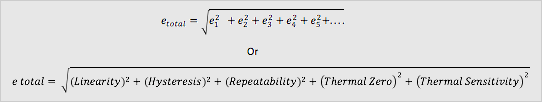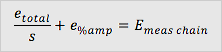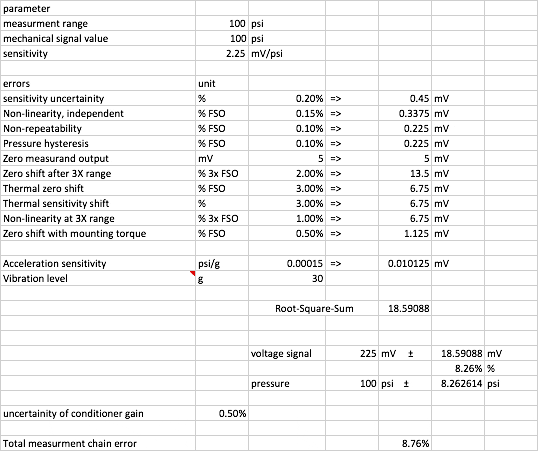### Question

Data Sheets of Endevco pressure transducers are detailed, identifying the contribution of various factors to the total measurement error. What I would like to know is the total error in the case of a measurement I am currently carrying out. So in other words: how can I calculate the total error of the transducer output based on the information given in calibration certificate and data sheet?

All error types presented in the typical data sheet are the systematic errors - so the errors related to the limited precision of the equipment are ones that cannot be estimated by repeating the measurement. Calculation of resultant total measurement error etotal is not as complex. The possible contribution of particular error sources ex has to be added together in root-square-sum, according the following formula:Before the calculations can be completed we have to make sure that all added components are expressed in the same unit (pC or mV), so that they are additive. Also some of the total error contributors will be dependent on the signal amplitude, the percentage of the transducer indication (e.g. amplitude nonlinearity) or in % of full-scale output (e.g. pressure hysteresis). Some errors can be expressed as an absolute value (e.g. ZMO, noise). In the case of a particular application, certain error sources may be neglected, as they can be irrelevant in given conditions.

For example, if the temperature is stable there is no need of taking the thermal stability into account; we just have to correct the sensitivity value at a given temperature. Such an approach cannot be applied in the case of applications where temperature changes dynamically and/or are not measured.

If before the measurement the pressure gauge is balanced at the atmospheric pressure there is no need of taking the ZMO related error into account. The same concerns also apply to other parameters like e.g. ZMO shift after 3x over ranging. If the sensor was not over ranged this parameter does not have to be taken into account. And so on...

There are other measurement error sources that can be related to performance of any particular conditioning system, like accuracy of gain or input or output noise. As the relation between the transducer output and conditioner gain is multiplicative, the easiest way of calculating the overall measurement chain error is to calculate the relative error of pressure transducer (absolute total error etotal [mV] divided by the signal value s (mV) ) output expressed in % and simply add the relative error of conditioner gain expressed in %.In all cases when the gain of the conditioner is adjusted, to provide optimal performance and maximum dynamic range. In most cases noise may be neglected as its contribution is small in comparison to other error sources.